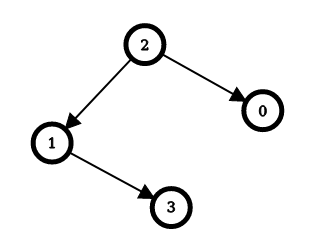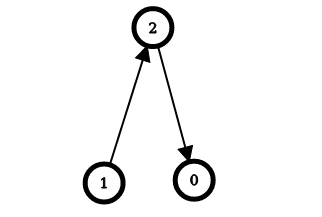September 25, 2023
Latest:
LeetCode Solution

# Minimum Edge Reversals So Every Node Is Reachable LeetCode Solution

### Minimum Edge Reversals So Every Node Is Reachable LeetCode Solution## Problem

### Minimum Edge Reversals So Every Node Is Reachable

• User Accepted:0
• User Tried:3
• Total Accepted:0
• Total Submissions:4
• Difficulty:Hard

There is a simple directed graph with `n` nodes labeled from `0` to `n - 1`. The graph would form a tree if its edges were bi-directional.

You are given an integer `n` and a 2D integer array `edges`, where `edges[i] = [ui, vi]` represents a directed edge going from node `ui` to node `vi`.

An edge reversal changes the direction of an edge, i.e., a directed edge going from node `ui` to node `vi` becomes a directed edge going from node `vi` to node `ui`.

For every node `i` in the range `[0, n - 1]`, your task is to independently calculate the minimum number of edge reversals required so it is possible to reach any other node starting from node `i` through a sequence of directed edges.

Return an integer array `answer`, where `answer[i]` is the minimum number of edge reversals required so it is possible to reach any other node starting from node `i` through a sequence of directed edges.

Example 1:```Input: n = 4, edges = [[2,0],[2,1],[1,3]]
Output: [1,1,0,2]
Explanation: The image above shows the graph formed by the edges.
For node 0: after reversing the edge [2,0], it is possible to reach any other node starting from node 0.
For node 1: after reversing the edge [2,1], it is possible to reach any other node starting from node 1.
For node 2: it is already possible to reach any other node starting from node 2.
For node 3: after reversing the edges [1,3] and [2,1], it is possible to reach any other node starting from node 3.
```

Example 2:```Input: n = 3, edges = [[1,2],[2,0]]
Output: [2,0,1]
Explanation: The image above shows the graph formed by the edges.
For node 0: after reversing the edges [2,0] and [1,2], it is possible to reach any other node starting from node 0.
For node 1: it is already possible to reach any other node starting from node 1.
For node 2: after reversing the edge [1, 2], it is possible to reach any other node starting from node 2.
```

Constraints:

• `2 <= n <= 105`
• `edges.length == n - 1`
• `edges[i].length == 2`
• `0 <= ui == edges[i] < n`
• `0 <= vi == edges[i] < n`
• `ui != vi`
• The input is generated such that if the edges were bi-directional, the graph would be a tree.